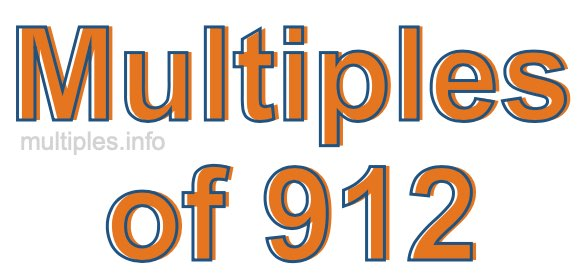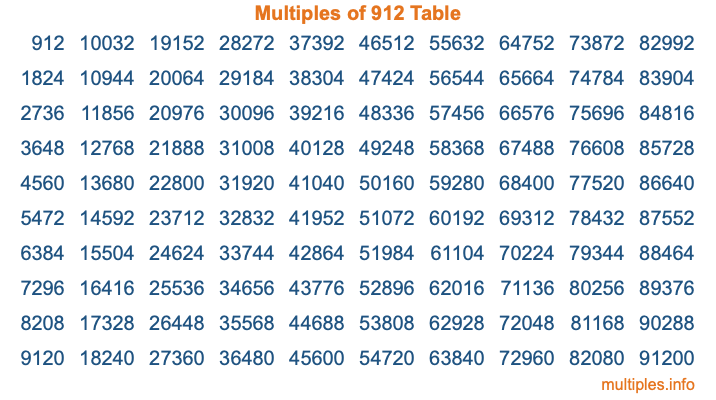Multiples of 912Welcome to the Multiples of 912 page. Here we will first teach you everything you will ever need to know about the multiples of 912, and then give you a study guide summary of everything we taught you to make sure you remember it all. Use this page to look up facts and learn information about the multiples of 912. This page will make you a multiples of nine hundred twelve expert!

Definition of Multiples of 912
Multiples of 912 are all the numbers that when divided by 912 equal an integer. Each of the multiples of 912 are called a multiple. A multiple of 912 is created by multiplying 912 by an integer.

Therefore, to create a list of multiples of 912, you start with 1 multiplied by 912, then 2 multiplied by 912, then 3 multiplied by 912, and so on for as long as you want. Thus, the list of the first five multiples of 912 is 912, 1824, 2736, 3648, and 4560. To see a larger list of multiples of 912, see the printable image of Multiples of 912 further down on this page. We also have a category where you can choose any nth multiple of 912.

Multiples of 912 Checker
The Multiples of 912 Checker below checks to see if any number of your choice is a multiple of 912. In other words, it checks to see if there is any number (integer) that when multiplied by 912 will equal your number. To do that, we divide your number by 912. If the the quotient is an integer, then your number is a multiple of 912.

Is  a multiple of 912?

Least Common Multiple of 912 and ...
A Least Common Multiple (LCM) is the lowest multiple that two or more numbers have in common. This is also called the smallest common multiple or lowest common multiple and is useful to know when you are adding our subtracting fractions. Enter one or more numbers below (912 is already entered) to find the LCM.

Check out our LCM Calculator if you need more details about the Least Common Multiple or if you need the LCM for different numbers for adding and subtraction fractions.

nth Multiple of 912
As we stated above, 912 is the first multiple of 912, 1824 is the second multiple of 912, 2736 is the third multiple of 912, and so on. Enter a number below to find the nth multiple of 912.

th multiple of 912

Multiples of 912 vs Factors of 912
912 is a multiple of 912 and a factor of 912, but that is where the similarities end. All postive multiples of 912 are 912 or greater than 912. All positive factors of 912 are 912 or less than 912.

Below is the beginning list of multiples of 912 and the factors of 912 so you can compare:

Multiples of 912: 912, 1824, 2736, 3648, 4560, etc.

Factors of 912: 1, 2, 3, 4, 6, 8, 12, 16, 19, 24, 38, 48, 57, 76, 114, 152, 228, 304, 456, 912

As you can see, the multiples of 912 are all the numbers that you can divide by 912 to get a whole number. The factors of 912, on the other hand, are all the whole numbers that you can multiply by another whole number to get 912.

It's also interesting to note that if a number (x) is a factor of 912, then 912 will also be a multiple of that number (x).

Multiples of 912 vs Divisors of 912
The divisors of 912 are all the integers that 912 can be divided by evenly. Below is a list of the divisors of 912.

Divisors of 912: 1, 2, 3, 4, 6, 8, 12, 16, 19, 24, 38, 48, 57, 76, 114, 152, 228, 304, 456, 912

The interesting thing to note here is that if you take any multiple of 912 and divide it by a divisor of 912, you will see that the quotient is an integer.

Multiples of 912 Table
Below is an image of the first 100 multiples of 912 in a table. The table is in chronological order, column by column. The first column has the first ten multiples of 912, the second column has the next ten multiples of 912, and so on.The Multiples of 912 Table is also referred to as the 912 Times Table or Times Table of 912. You are welcome to print out our table for your studies.

Negative Multiples of 912
Although not often discussed or needed in math, it is worth mentioning that you can make a list of negative multiples of 912 by multiplying 912 by -1, then by -2, then by -3, and so on, to get the following list of negative multiples of 912:

-912, -1824, -2736, -3648, -4560, etc.

Multiples of 912 Summary
Below is a summary of important Multiples of 912 facts that we have discussed on this page. To retain the knowledge on this page, we recommend that you read through the summary and explain to yourself or a study partner why they hold true.

There are an infinite number of multiples of 912.

A multiple of 912 divided by 912 will equal a whole number.

912 divided by a factor of 912 equals a divisor of 912.

The nth multiple of 912 is n times 912.

The largest factor of 912 is equal to the first positive multiple of 912.

912 is a multiple of every factor of 912.

912 is a multiple of 912.

A multiple of 912 divided by a divisor of 912 equals an integer.

912 divided by a divisor of 912 equals a factor of 912.

Any integer times 912 will equal a multiple of 912.

Multiples of a Number
Here you can get the multiples of another number, all with the same attention to detail as we did for multiples of 912 on this page.

Multiples of
Multiples of 913
Did you find our page about multiples of nine hundred twelve educational? Do you want more knowledge? Check out the multiples of the next number on our list!